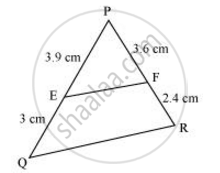# E and F are points on the sides PQ and PR respectively of a ΔPQR. For the following case, state whether EF || QR. PE = 3.9 cm, EQ = 3 cm, PF = 3.6 cm and FR = 2.4 cm - Mathematics

E and F are points on the sides PQ and PR respectively of a ΔPQR. For the following case, state whether EF || QR. PE = 3.9 cm, EQ = 3 cm, PF = 3.6 cm and FR = 2.4 cm

#### SolutionGiven that, PE = 3.9 cm, EQ = 3 cm, PF = 3.6 cm, FR = 2.4 cm

(PE)/(EQ)=(3.9)/3 = 1.3

(PF)/(FR) = 3.6/2.4 = 1.5

Hence, (PE)/(EQ) != (PF)/(FR)

Therefore, EF is not parallel to QR

Concept: Similarity of Triangles
Is there an error in this question or solution?

#### APPEARS IN

NCERT Class 10 Maths
Chapter 6 Triangles
Exercise 6.2 | Q 2.1 | Page 128